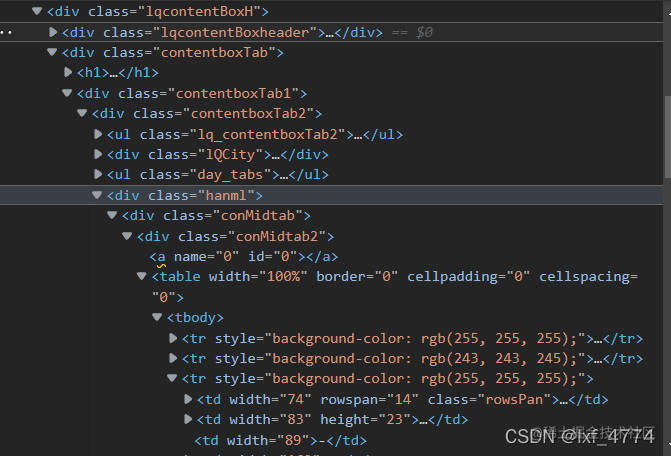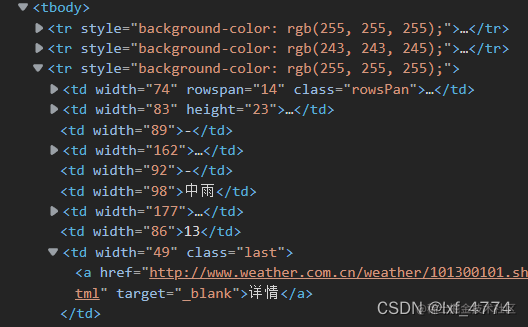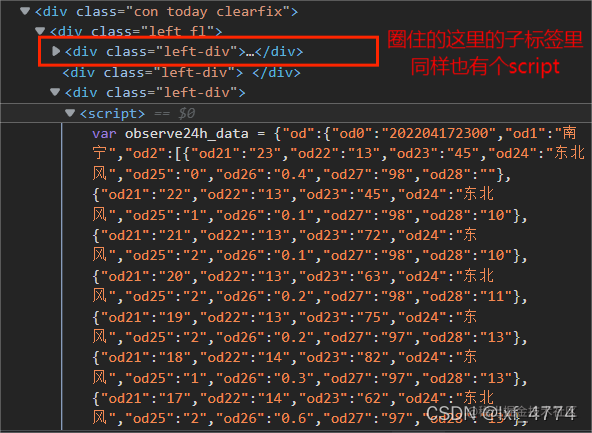# 遇到的问题：json解析之后，如何得到相应的json的值，并进行临时储存

# 解决方法：

```python def get_html(self, url): # 设置标头 headers = { 'User-Agent': 'Mozilla/5.0 (Windows NT 10.0; Win64; x64) AppleWebKit/537.36 (KHTML, like Gecko) Chrome/99.0.4844.74 Safari/537.36' } # 得到get请求的回应 response = requests.get(url, headers=headers) # 进行文本编码，去除乱码情况 html = response.text.encode('iso-8859-1').decode('utf-8') # 返回源代码 return html``` 对于第二个问题，正则表达式需要一一匹配，有点过于繁琐，所以我们采用了PyQuery库来进行解析，快速且易读，并且，由于我们的链接在多个相同结构的标签里面，那么用正则匹配出来的是字符串，可能是连在一起，不好分离。而PyQuery库中，我们可以使用items()函数生成一个生成器，通过遍历生成器里的内容，可以单独地获取我们需要的每一个城市的网址。 代码如下：

```python def get_new_url(self, html): # 将网页源代码转换成pq对象 html = pq(html) # 获取具体网址的生成器，其中每个生成器内的子器代表着一个城市的超链接 items = html('div.lqcontentBoxH>div.contentboxTab>div.contentboxTab1>div.contentboxTab2>div.hanml>div.conMidtab>div.conMidtab2>table>tr>td.last>a').items() # 储存网址 new_url = [] # 获取href属性中的内容 for item in items: new_url.append(item.attr('href')) # 返回列表类型的超链接 return new_url``` 对于第三个问题，因为使用PyQuery库进行解析不太方便，我们就不再使用pq库来解析了，而我们以解决1中的代码可以得到一个response.text进行过编码后的网页源代码，其类型如text所言，是一个字符串类型。我们知道，字符串类型用有一个find方法可以帮我们快速找到我们指定的字符串内容是否在其之中，存在时会返回第一个字符的索引值。由此，我们可以使用find方法来帮我们快速定位所找json字符串的位置；然后对于script标签的储存的动态数据，其内容是多层字典的形式，我们可以使用json.loads方法对其进行解析，将字符串类型转换成我们想要的字典类型，代码如下：

```python

# 找到od2后的下一层的具体数据

``````    text = js_['od']['od2']
# 找到od1中储存的城市名
city = js_['od']['od1']
# 临时储存天气数据(创建数表)，查看了一下数据，除了od23不知道是什么数据，其他的都可以知道，因此在设置列索引时，我们就直接进行了修改，方便我们后续查看
temp_weather = pd.DataFrame([], columns=['时间', '温度', 'od23', '风向', '风力', '降水量', '相对湿度', '空气质量'],index=[x+1 for x in range(len(text)-1)])
# 写入数据
for i in range(len(text)):
# 为什么需要跳过这个呢？这是因为od2中储存的其实有25组数据，即25个小时的数据，即会有一个是两天的同一个时辰的数据，后面画图会有些麻烦，这里为了避归这一麻烦，且第二天同一时辰的数据对后面也没有太多的影响，就选择跳过这一问题了
if i == 0:
continue
# 写入时间，没有进行类型变化，因此时间是字符串类型，而这里的横作为有23开始是因为其字典的顺序本身就是反过来的
temp_weather.iloc[23-i, 0] = text[i]['od21']
# 写入温度，转换成float类型
temp_weather.iloc[23-i, 1] = float(text[i]['od22'])
# od23不清楚指的是什么内容，但对本爬虫的要求无影响，故不做处理，之后会进行删除处理
temp_weather.iloc[23-i, 2] = text[i]['od23']
# 写入风向，字符串类型
temp_weather.iloc[23-i, 3] = text[i]['od24']
# 写入风力，由于不做要求，因此不做任何处理，后续会进行删除处理
temp_weather.iloc[23-i, 4] = text[i]['od25']
# 写入降水量，转换成浮点型
temp_weather.iloc[23-i, 5] = float(text[i]['od26'])
# 写入相对湿度，转换成浮点型
temp_weather.iloc[23-i, 6] = float(text[i]['od27'])
# 写入空气质量，由于最后一处出现缺失（空值），目前还转换不了浮点型，后面会进行处理
temp_weather.iloc[23-i, 7] = text[i]['od28']
# 得到数表后，将od23和风向风力的数据进行删除
temp_weather.drop(labels=['od23', '风向', '风力'], axis=1, inplace=True)
# 将那个字符串空值赋予缺失值处理
temp_weather.iloc[23, 4] = np.nan
# 将空气质量的类型由字符串转换成浮点型
temp_weather['空气质量'] = temp_weather['空气质量'].astype('float')
# 然后将刚刚那个缺失值进行处理，这里使用的是前填充，使其与上一个小时的AQI值保持一致
temp_weather.fillna(method='ffill', inplace=True)
``````

```

```Python def printing(self, df): # 保持时间（为字符串类型，这样子就不会在后续的图片中出现matplotlib自动将数值进行排序从0开始的情况，使之从今时开始） x = list(df.iloc[:, 0]) # 依次储存温度、降水量、相对湿度、空气质量AOI的值，转换成列表类型 temperature = list(df.iloc[:, 1]) rainfall = list(df.iloc[:, 2]) humidity = list(df.iloc[:, 3]) air_quality = list(df.iloc[:, 4]) plt.figure(figsize=(20, 10), dpi=80) plt.subplot(221) # AQI图 # 储存AQI最大值 max_air = max(air_quality) # 画出柱形图 plt.bar(x, air_quality, color='pink', label='空气质量(AQI)') # 设置横纵坐标轴的标签--对应显示的内容的含义 plt.ylabel('数值') plt.xlabel('时间/h') # 设置图片标题 plt.title(f'{city}市24h内空气质量变化示意图') # 设置文本框 plt.text(x=float(min(x)), y=max_air + 0.8, s=f'24h内AQI最高值为{max_air}', color='white', bbox={ 'color': 'gray', 'alpha': 0.5 }) # 显示出每条柱对应的值 for i in range(len(air_quality)): plt.text(x[i], air_quality[i], '%.0f' % air_quality[i], ha='center', va='bottom', color='#74C476') # 设置网格，仅设置y轴上的横线 plt.grid(alpha=1, axis='y', linestyle='--') # 设置图例 plt.legend(loc='upper right')

``````plt.subplot(222)
# 相对湿度图
# 储存最大相对湿度
max_humidty = max(humidity)
# 画出折线图以显示其变化
plt.plot(x, humidity, color='#74C476', label='相对湿度')
# 标出各点
plt.scatter(x, humidity, color='r')
# 设置横纵坐标标签，横轴表示时间，纵轴表示相对湿度的大小
plt.ylabel('相对湿度/%')
plt.xlabel('时间/h')
# 设置图片标题
plt.title(f'{city}市24h内空气相对湿度变化图')
# 设置文本框，对图片进行适当说明
plt.text(x=int(min(x)), y=max_humidty - 0.4, s=f'24h内最高相对湿度为{max_humidty}%', color='white',
bbox={
'color': 'gray',
'alpha': 0.5
})
# 设置图例位置
plt.legend(loc='upper center'）

# 降水量图
plt.subplot(223)
# 依次储存总降水量、最小/大降水量
sum_rain = round(sum(rainfall), 2)
min_rain = min(rainfall)
max_rain = max(rainfall)
# 用柱形图画出降水量情况
plt.bar(x, rainfall, color='pink', label='降水量')
# 设置横纵坐标轴的含义，横轴表示时间，纵轴表示降水量
plt.xlabel('时间/h')
plt.ylabel('降水量/mm')
# 设置图片标题
plt.title(f'{city}市24h内降水量示意图')
# 显示出每条柱对应的值
for i in range(len(rainfall)):
plt.text(x[i], rainfall[i], '%.1f' % rainfall[i], ha='center', va='bottom', color='#74C476')
# 在图片中设置文本，内容包含降水极值的说明
# annotate可在样本点附近进行说明，但是不太美观
# text自己设置位置放置，统一说明，可以加背景框，显得好看点，芜湖，x、y为横、纵坐标，s输入所需显示的文字，调色，bbox是设置背景框用的
plt.text(x=int(min(x)), y=max_rain - 0.4, s=f'最高降水量为{max_rain}mm\n最低降水量为{min_rain}mm\n总降水量为{sum_rain}mm',
color='white',
bbox={
'color': 'gray',
'alpha': 0.5
})
# 设置网格线
plt.grid(alpha=1, axis='y')
# 设置图例内容
plt.legend(loc='upper right')

# 气温图
# 储存最高/低气温值
plt.subplot(224)
max_tem = max(temperature)
min_tem = min(temperature)
# 画出温度变化图
plt.plot(x, temperature, color='#74C476', label='温度')
# 标出各时间段的气温点位置
plt.scatter(x, temperature, color='blue')
# 设置横纵坐标轴的含义，横轴表示时间，纵轴表示气温
plt.xlabel('时间/h')
plt.ylabel('温度/℃')
# 设置图片标题
plt.title(f'{city}市24h温度变化示意图')
# 设置文本框，显示适当的文字说明以还原图像
plt.text(x=x, y=max_tem - 0.3, s=f'最高气温为{max_tem}ml\n最低气温为{min_tem}ml', color='white',
bbox={
'color': 'gray',
'alpha': 0.5
})
# 设置图例位置
plt.legend(loc='upper right')
plt.show()
``````

```

# 全部代码如下：

```python import json import pandas as pd import requests from pyquery import PyQuery as pq from matplotlib import pyplot as plt import matplotlib import numpy as np

# 在图片中显示中文

matplotlib.rc("font", family="MicroSoft YaHei", weight='bold', size=13)

class Weather(object): def get_html(self, url): # 设置标头 headers = { 'User-Agent': 'Mozilla/5.0 (Windows NT 10.0; Win64; x64) AppleWebKit/537.36 (KHTML, like Gecko) Chrome/99.0.4844.74 Safari/537.36' } # 得到get请求的回应 response = requests.get(url, headers=headers) # 进行文本编码，去除乱码情况 html = response.text.encode('iso-8859-1').decode('utf-8') # 返回源代码 return html

``````def get_new_url(self, html):
# 将网页源代码转换成pq对象
html = pq(html)
# 获取具体网址的生成器，其中每个生成器内的子器代表着一个城市的超链接
items = html('div.lqcontentBoxH>div.contentboxTab>div.contentboxTab1>div.contentboxTab2>div.hanml>div.conMidtab>div.conMidtab2>table>tr>td.last>a').items()
# 储存网址
new_url = []
# 获取href属性中的内容
for item in items:
new_url.append(item.attr('href'))
# 返回列表类型的超链接
return new_url

def gain_data(self, new_html):
# 寻找数据开头位置
n = new_html.find('observe24h_data')
# 末尾位置
m = new_html.find('}]}}')
# 筛选
text = new_html[n+18:m+4]
# 返回筛选后的代码数据（字符串类型）
return text

def printing(self, df):
# 保持时间（为字符串类型，这样子就不会在后续的图片中出现matplotlib自动将数值进行排序从0开始的情况，使之从今时开始）
x = list(df.iloc[:, 0])
# 依次储存温度、降水量、相对湿度、空气质量AOI的值，转换成列表类型
temperature = list(df.iloc[:, 1])
rainfall = list(df.iloc[:, 2])
humidity = list(df.iloc[:, 3])
air_quality = list(df.iloc[:, 4])
# 设置整个的图纸大小
plt.figure(figsize=(20, 10), dpi=80)

plt.subplot(221)
# AQI图
# 储存AQI的最大值
max_air = max(air_quality)
# 画出柱形图
plt.bar(x, air_quality, color='pink', label='空气质量(AQI)')
# 设置横纵坐标轴的标签--对应显示的内容的含义
plt.ylabel('数值')
plt.xlabel('时间/h')
# 设置图片标题
plt.title(f'{city}市24h内空气质量变化示意图')
# 设置文本框
plt.text(x=float(min(x)), y=max_air + 0.8, s=f'24h内AQI最高值为{max_air}', color='white',
bbox={
'color': 'gray',
'alpha': 0.5
})
# 显示出每条柱对应的值
for i in range(len(air_quality)):
plt.text(x[i], air_quality[i], '%.0f' % air_quality[i], ha='center', va='bottom', color='#74C476')
# 设置网格，仅设置y轴上的横线
plt.grid(alpha=1, axis='y', linestyle='--')
# 设置图例
plt.legend(loc='upper right')

plt.subplot(222)
# 相对湿度图
# 储存最大相对湿度
max_humidty = max(humidity)
# 画出折线图以显示其变化
plt.plot(x, humidity, color='#74C476', label='相对湿度')
# 标出各点
plt.scatter(x, humidity, color='r')
# 设置横纵坐标标签，横轴表示时间，纵轴表示相对湿度的大小
plt.ylabel('相对湿度/%')
plt.xlabel('时间/h')
# 设置图片标题
plt.title(f'{city}市24h内空气相对湿度变化图')
# 设置文本框，对图片进行适当说明
plt.text(x=int(min(x)), y=max_humidty - 0.4, s=f'24h内最高相对湿度为{max_humidty}%', color='white',
bbox={
'color': 'gray',
'alpha': 0.5
})
# 设置图例位置
plt.legend(loc='upper center')

# 降水量图
plt.subplot(223)
# 依次储存总降水量、最小/大降水量
sum_rain = round(sum(rainfall), 2)
min_rain = min(rainfall)
max_rain = max(rainfall)
# 用柱形图画出降水量情况
plt.bar(x, rainfall, color='pink', label='降水量')
# 设置横纵坐标轴的含义，横轴表示时间，纵轴表示降水量
plt.xlabel('时间/h')
plt.ylabel('降水量/mm')
# 设置图片标题
plt.title(f'{city}市24h内降水量示意图')
# 显示出每条柱对应的值
for i in range(len(rainfall)):
plt.text(x[i], rainfall[i], '%.1f' % rainfall[i], ha='center', va='bottom', color='#74C476')
# 在图片中设置文本，内容包含降水极值的说明
# annotate可在样本点附近进行说明，但是不太美观
# text自己设置位置放置，统一说明，可以加背景框，显得好看点，芜湖，x、y为横、纵坐标，s输入所需显示的文字，调色，bbox是设置背景框用的
plt.text(x=int(min(x)), y=max_rain - 0.4, s=f'最高降水量为{max_rain}mm\n最低降水量为{min_rain}mm\n总降水量为{sum_rain}mm',
color='white',
bbox={
'color': 'gray',
'alpha': 0.5
})
# 设置网格线
plt.grid(alpha=1, axis='y')
# 设置图例内容
plt.legend(loc='upper right')

# 气温图
# 储存最高/低气温值
plt.subplot(224)
max_tem = max(temperature)
min_tem = min(temperature)
# 画出温度变化图
plt.plot(x, temperature, color='#74C476', label='温度')
# 标出各时间段的气温点位置
plt.scatter(x, temperature, color='blue')
# 设置横纵坐标轴的含义，横轴表示时间，纵轴表示气温
plt.xlabel('时间/h')
plt.ylabel('温度/℃')
# 设置图片标题
plt.title(f'{city}市24h温度变化示意图')
# 设置文本框，显示适当的文字说明以还原图像
plt.text(x=x, y=max_tem - 0.3, s=f'最高气温为{max_tem}ml\n最低气温为{min_tem}ml', color='white',
bbox={
'color': 'gray',
'alpha': 0.5
})
# 设置图例位置
plt.legend(loc='upper right')
plt.show()
``````

if name == 'main': # 爬取的网址 url = 'http://www.weather.com.cn/textFC/hn.shtml' # 创建天气爬虫对象 national_w = Weather() # 得到主页的html源代码 html = national_w.get_html(url) # 获取该地区（华南、华北之类的）的每个城市的天气预报网址 new_url = national_w.get_new_url(html) n = int(input(f'请输入要查询的城市数量(不大于{len(new_url)}的正整数)：')) for cnt in range(n): # 获取具体城市的天气预报的源代码 new_html = national_w.get_html(new_url[cnt]) # 获取script标签里面的数据 datas = national_w.gain_data(new_html) # 我们所需要的数据在script标签里面的var变量里，内部是多层的字典类型，因此，我们需要进行json解析转化成字典类型 js_ = json.loads(datas) # 找到od2后的下一层的具体数据 text = js_['od']['od2'] # 找到od1中储存的城市名 city = js_['od']['od1'] # 临时储存天气数据(创建数表)，查看了一下数据，除了od23不知道是什么数据，其他的都可以知道， # 因此在设置列索引时，我们就直接进行了修改，方便我们后续查看 temp_weather = pd.DataFrame([], columns=['时间', '温度', 'od23', '风向', '风力', '降水量', '相对湿度', '空气质量'], index=[x+1 for x in range(len(text)-1)]) # 写入数据 for i in range(len(text)): # 为什么需要跳过这个呢？这是因为od2中储存的其实有25组数据，即25个小时的数据， # 即会有一个是两天的同一个时辰的数据，后面画图会有些麻烦，这里为了避归这一麻烦， # 且第二天同一时辰的数据对后面也没有太多的影响，就选择跳过这一问题了 if i == 0: continue # 写入时间，没有进行类型变化，因此时间是字符串类型， # 而这里的横作为有23开始是因为其字典的顺序本身就是反过来的 temp_weather.iloc[23-i, 0] = text[i]['od21'] # 写入温度，转换成float类型 temp_weather.iloc[23-i, 1] = float(text[i]['od22']) # od23不清楚指的是什么内容，但对本爬虫的要求无影响，故不做处理，之后会进行删除处理 temp_weather.iloc[23-i, 2] = text[i]['od23'] # 写入风向，字符串类型 temp_weather.iloc[23-i, 3] = text[i]['od24'] # 写入风力，由于不做要求，因此不做任何处理，后续会进行删除处理 temp_weather.iloc[23-i, 4] = text[i]['od25'] # 写入降水量，转换成浮点型 temp_weather.iloc[23-i, 5] = float(text[i]['od26']) # 写入相对湿度，转换成浮点型 temp_weather.iloc[23-i, 6] = float(text[i]['od27']) # 写入空气质量，由于最后一处出现缺失（空值），目前还转换不了浮点型，后面会进行处理 temp_weather.iloc[23-i, 7] = text[i]['od28'] # 得到数表后，将od23和风向风力的数据进行删除 temp_weather.drop(labels=['od23', '风向', '风力'], axis=1, inplace=True) # 将那个字符串空值赋予缺失值处理 temp_weather.iloc[23, 4] = np.nan # 将空气质量的类型由字符串转换成浮点型 temp_weather['空气质量'] = temp_weather['空气质量'].astype('float') # 然后将刚刚那个缺失值进行处理，这里使用的是前填充，使其与上一个小时的AQI值保持一致 temp_weather.fillna(method='ffill', inplace=True) # 开始绘制图像，依次为温度、降水量、相对湿度、空气质量AOI的变化示意图 national_w.printing(temp_weather) ```

# 补充：

plt.text：添加文本，可以自由的设置文本出现的位置，参数s的作用是给我们输入自己自定义的字符串进行显示。同时还有一个bbox参数，使我们可以设置一个文本框，给文本大致划个范围且醒目。与此同时，我们可以在画柱形图的时候采用text方法来对每个柱形上面添加其值的大小的文本，使图片可观性、易读性更好

```python plt.text(x=0,#文本x轴坐标 y=0, #文本y轴坐标 s='basic unility of text', #文本内容

``````     # 依次设置的是字体大小，颜色和字体种类
fontdict=dict(fontsize=12, color='r',family='monospace',),#字体属性字典

#添加文字背景色
bbox={'facecolor': 'red', #填充色
'edgecolor':'y',#外框色
'alpha': 0.5, #框透明度，值越大越不透明
'boxstyle':'sawtooth'
}

)
``````

```

plt.annotate：也是添置文本，与text不同的是，我们可以加入一个参数arrowprops使其可以显示一个箭头指向所需解释的点，实现了点与文本分离，但个人感觉有点不太美观啦

```python plt.annotate('basic unility of annotate', xy=(x, y),#箭头末端位置

``````         xytext=(x1, y1),#文本起始位置

#箭头属性设置
arrowprops=dict(facecolor='red', # 箭头颜色
shrink=1,#箭头的收缩比
alpha=0.5,#透明度
width=7,#箭身宽
hatch='--',#填充形状
frac=0.8,#身与头比
#其它参考matplotlib.patches.Polygon中任何参数
),
)
``````

```

「其他文章」